# 2 Mathematics without numbers

It is true that thousands of years ago, humanity began to conceive the idea of numbers and began to do calculations, until 2500 years ago, some philosophers began to wonder about the numbers themselves, apart from practical usage. They invented the axiomatic deductive method and established thus mathematics as an exact science. Philosophy (love of wisdom) had thus come across a field, where you can penetrate to the truth. Every cheeky assertion or assumption must be proved or can be disproved. But it was only in the twentieth century, that mathematicians succeeded in bringing the by then scattered results under one roof and onto common foundations. This is sometimes referred to as the modern mathematics; Adler . Since then, the lectures for beginners at university are not about numbers, it's all about propositions and how to handle them. We have even to pretend to be creatures, who are utterly ignorant of numbers, but who are about to discover operations with certain things.
During your first weeks at university this may be bothersome for you. But make the best of it and internalize this mode of thinking, if possible, well in advance.
When I was in this situation, there was a blind student among us. Instead of doing nothing after leaving school, he had worked hard, so as not to fall behind on account of his handicap. Once in a while, lecturers would ask him, if he is able to follow an argument. Unerringly, he used to answer: "I can see very clearly". Quite often, the majority of us was irritated, because we were in the dark but didn't have the guts to ask. Most lecturers like it, when you come forward with questions.

With "P := Today is Sunday", we define P to mean a whole sentence, in order to make our work easy and more lucid. For doing so, we use another abbreviation := (conceptualization). Also =: is in use; the colon is next to what is being defined. We learn here also, that a proposition needs not absolutely to be true or false. It may depend on circumstances.

# 3 Proposition, truth-value, equivalence

In mathematics, propositions are either true (T) or false (F) and the truth or falsity of each proposition is said to be its truth-value. The negation of a proposition (we use the operator ¬) switches the truth-value . If P is the proposition "a ≠ b", then ¬P is the proposition "a = b".
A compound proposition is an assembly of statements which are connected by sentential connectives (logical connectives) like the conjunction ∧ (AND-operation) and the disjunction ∨ (OR-operation).
If the proposition P implies the proposition Q, (implication), we write P ⇒ Q (or Q ⇐ P). Hold both, P ⇒ Q and Q ⇒ P, we write P ⇔ Q (equivalence).
So as to save even more space, ink and energy when writing statements, we use the energy-saving quantifiers ∀ ("for all") and ∃ ("there exists") that indicate the scope of a term to which it is attached. (Sometimes we may come across ∃! := "there exist exactly one").
A truth table is a very helpful aid for evaluating a compound proposition. We draw a chart that shows in the rightmost column the compound proposition we are interested in. In the leftmost columns we write the propositions that shall make up the compound one. In between the leftmost columns and the rightmost column we provide columns for the intermediate results that we need in order to find the compound proposition we are looking for. We start by writing all possible truth-values in the lefthand columns and then work our way from left to right.
Example of a truth table: For two propositions P and Q we put in the lefthand columns the possible combinations of truth-values. In this case, there are four possibilities and thus four rows to the table.
 P Q ¬P ¬Q P ∨ ¬Q ¬P ∨ ¬Q (P ∨ ¬Q) ∧ ( ¬P ∨ ¬Q) T T F F T F F T F F T T T T F T T F F T F F F T T T T T

# 4 Sets and Subsets

So far we have not yet defined any number system, but we know that it won't be long; also we know from school, that we are going to handle sets of numbers.
In mathematics, if we make use of a concept that hasn't been defined yet, we say that we use the concept naively. We still use the different number concepts naively. As far as the concept of a set is concerned, we leave it at the naive use, because the theory of sets is an axiomatic deductive theory in itself (Halmos [26, 26a], Ebbinghaus ), to which you can devote yourself fully, if you are interested, when you have climbed the astonishing stairs of the basics and can have a look around in the big reception hall of the mathematical building.

By a set S we mean an object that is a collection of well-distinguished objects, which need not be arranged and which are called the elements of S.
With { } we symbolize a container which we call the empty set, a set, which doesn't contain any element. For the non-empty sets M := {a, b, a,} and {b, a} =: N, M = N is a true proposition, because every element counts only once, and the order is not relevant. We have a ∈ N (a is an element of N) and c ∉ M (c is not an element of M).

We use Venn diagrams in order to visualize operations with sets, as shown in Abb 1 to 6. (We don't use diagrams to define or prove anything).
With C := A ∩ B  = {x | x ∈ A ∧ x ∈ B} we assign C the intersection of the sets A and B (Abb 1).
If, as result of this operation, C is not empty, it contains all those elements that A and B have in common (Abb 1).
If C is empty, we say that A and B are disjoint (Abb 3).
If however A ∩ B = B holds, we write B ⊂ A (inclusion) and call B a subset of A (Abb 2) or we say B is contained in A.
If both B ⊂ A and A ⊂ B hold, then A = B holds.
Since { } contains no element which is not contained in every other set, we have { } ⊂ M for every set M.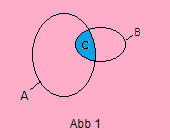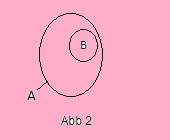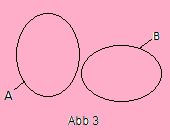In order to obtain the union of two sets A and B, we empty them into one bag, discard with duplicates and write A ∪ B  (Abb 6). Therefore A ∪ A = {x | x ∈ A ∨ x ∈ A} = A (not 2A).
If a set B doesn't want to have something in common with a set A, it removes its intersection with A and calls himself from now on B - A, the relative complement of A (Abb 4).
The set A ⊕ B := (A - B) ∪ (B - A) = (A ∪ B)  -  (A ∩ B) is called the disjoint union of A and B; see (Abb 5).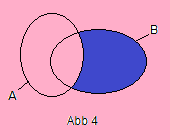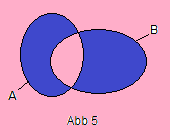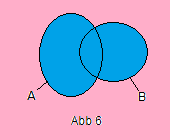The following notations are reserved for standard sets:
∅ := { }   (the empty set)
N := the set of natural numbers = {1, 2, 3, ...}
N0 := {0, 1, 2, 3, ⋅⋅⋅}
Z := -N ∪ 0 ∪ N = {⋅⋅⋅, -3, -2, -1, 0, 1, 2, 3, ⋅⋅⋅}     (the set of integers)
Q := {p∕q | p, q ϵ Z ∧ q ≠ 0}, the set of rational numbers = all (proper, terminated and all periodic infinite) fractions
R := the set of real numbers
C := the set of complex numbers, where C = {(a + ib) | a,b ∈ R, i² = -1}
We have: ∅ ⊂ NN0ZQRC
With |M| we denote the cardinality of the set M. For a set M having a finite number n of elements, we write |M| = n, for a set M having an infinite number of elements, we write |M| = ∞. (Cantorsche Kontinuumshypothese: CH ("Continuum Hypothesis") : 20 = ℵ1).
If for sets M and A, A ⊂ M holds, we call B := M - A the complement of A (in M). For example, the even numbers form the complement of the uneven numbers (in the set of the integers Z).

### The power set of a set

We can also form sets whose elements are sets. But be careful to avoid sets that contain themselves, or similar nonsense. The power set P(M) of a set M is the set of all subsets of M, including Ø and M. If M := {a, b, c}, then P(M) = {Ø, {a}, {b}, {c}, {a, b}, {a, c}, {b, c}, M}. For a finite set M,

`|P(M)| = 2|M|.`

### Set products (Cartesian products)

Let A1, A2, ... ,An be sets. The product of these sets is written A1 x A2 x ... x An. this is a new set, also called the Cartesian product of the Ai and the elements of this new set are called n-tupels which are of the form (a1, a2, ... ,an), where ai ∈ Ai. If A1 has n1 elements, there are n1 possibilities to occupy the first place in the n-tupel. For the second position there are n2 possibilities ... , for the last position there are nn possibilities. It is now easy to see, that |A1 x A2 x ... x An| = |A1| ⋅ | A2| ⋅ ... ⋅ |An|.
An example for Ai = Aj ist R2 := R x R =: C. The elements of C are the complex numbers (a, b). The first position in this 2-tupel is occupied by the real part, the second position by the imaginary part. The number (a, b) is also written as a + ib.
R x R is a fine example for the fact, that a set becomes only "useful", when we provide it with operations and axioms. In the shape of C, R2 constitutes the complex numbers in the Gaussian number plane, in analytical geometry of the plane it is the set of coordinates of the points of the plane and in a 2-dimensional vector space it serves as set of elements of the space.

# 5 Relations

A relation R between two sets A and B is a subset of the Cartesian product A x B. If A has m elements and B has n elements, then |A x B| = m · n. Therefore, a relation (subset) of A x B can have at most |A x B| elements. The elements are 2-tuples, i. e. ordered pairs (a, b) with a ∈ A und b ∈ B. The set A containing the left components is called the domain and the set B containing the right components is called the codomain of R. The 2-tuple (a, b) may not be confused with the set {a, b} = {b, a}. A short way to indicate that (a, b) ∈ R is aRb. We usually give a relation a name which signifies the relationship in which we are interested. If I define M : = "is married to", I can in future write JohnMNelly, instead of "John (from A) is married to Nelly (from B).
The Cartesian product A x B depicted in Abb 7 has 20 elements. Each subset of it represents a relation. Let R be the relation {(e, 3), (c, 2), (a, 4), then we have for example (b, 1) ∉ R but (e, 3) ∈ R. Let A := the set of the inhabitants of Kremsmünster, B := the set of the inhabitants of Buxtehude, and L the relationen "is in love with". Then, as far as I know, there is only one element in this relation A x B. Franz from Kremsmünster is in love with Marion from Buxtehude. From this relation,{(Franz, Marion)} we are not able to tell, if Marion is in love with Franz too (symmetry), or whether Marion loves Karl and therefore, Franz also loves Karl (transitivity) ore if Franz loves himself (reflexivity). Properties of this kind can only be found in Cartesian products A x A, like for example in R2. Therefore, the most important case is of a relation R between S and itself. This is called a relation on the set S (i. e. R ⊂ S x S).

# 6 Relational structures

A set together with one or more relations defined on it is called a relational (algebraic) structure.

Equivalence relation on a set and partion of a set
If S is a set and R is a relation on S, then R is said to be an equivalence relation ⇔
a) x ∈ S ⇒ xRx
b) x, y ∈ S and xRy ⇒ yRx
c) x, y, z ∈ S and xRy and yRz ⇒ xRz
The properties a, b, c are called the reflexive, symmetric and transitive properties, respectively. A popular label for equivalence relations is ∼. An equivalence relation always goes along with a partition (decomposition) of the supporting set S into disjoint subsets, the equivalence classes [a] = {x ∈ S | x∼a} of ∼. The set of equivalence classes C| = {[x] | x ∈ S} has thus the same elements as S, only grouped into equivalence classes. Example: the set of the even and the set of the odd numbers are disjoint and their union gives the set of the integers. In this relation, the even numbers have nothing to do with the uneven numbers and vice versa (ei∼ej, ei≁oj, oi∼oj, oi≁ej).
Partial orders
are relations which are reflexive, antisymmetric and transitive and for aRb we write a ≼ b. For example in the power set P(M) = {{ }, {a}, {b}, {c}, {a, b}, {a, c}, {b, c}, M} of the set M = {a, b, c}, the elements of the sequence ({ }, {b}, {b, c}, {a, b, c}) are comparable in this way. In the Hasse diagram in Abb 8 you can detect other sequences.

Well orders
are relations which are irreflexive, antisymmetric and transitive and for aRb we write a ≺ b. In this case, any two elements of the supporting set are comparable.

# 7 The theories of Groups, Rings, Fields

The concept of a Group
A group (G, ∘) is a nonempty set G together with a binary operation ∘ defined on G such that the following axioms are satisfied:
G1. Associative Law. a, b, c ∈ G ⇒ a ∘ (b ∘ c) = (a ∘ b) ∘ c
G2. Identity. ∃ e ∈ G | e ∘ x = x ∘ e = x
G3. Inverse. ∀ x ∈ G ∃ y ∈ G | x ∘ y = y ∘ x = e.
(G, ∘) is commutative (abelian) ⇔ a, b ∈ G ⇒ a ∘ b = b ∘ a.
The concept of a Ring
A Ring (R, +, ⋅) is a nonempty set R together with two binary operations + and ⋅ (addition and multiplication) defined on G such that the following axioms are satisfied:
R1. (R, +) is an abelian Group
R2. Multiplication is associative
R3. The following distributive laws are satisfied:
For all a, b, c ∈ R
a ⋅ (b + c) = a ⋅ b + a ⋅ c, (b + c) ⋅ a = b ⋅ a + c ⋅ a
A ring in which the multiplication is commutative is a commutative ring. A ring with a multiplicative identity element is a ring with unity (unitary ring). The zero ring ({0}, +, ⋅) is also called the trivial ring.
The concept of a Field
A Field is a nontrivial, commutative ring with unity (multiplicative identity element) in which every nonzero element is a unit (has an inverse with respect to multiplication). The structure ({0, 1}, +, ⋅) is the smallest possible field (here 1 + 1 = 0). The sets Q, R and C make up the most familiar fields.

• 8 The real numbers R    >    | Chapter 8 |

•
• Schreib mir was